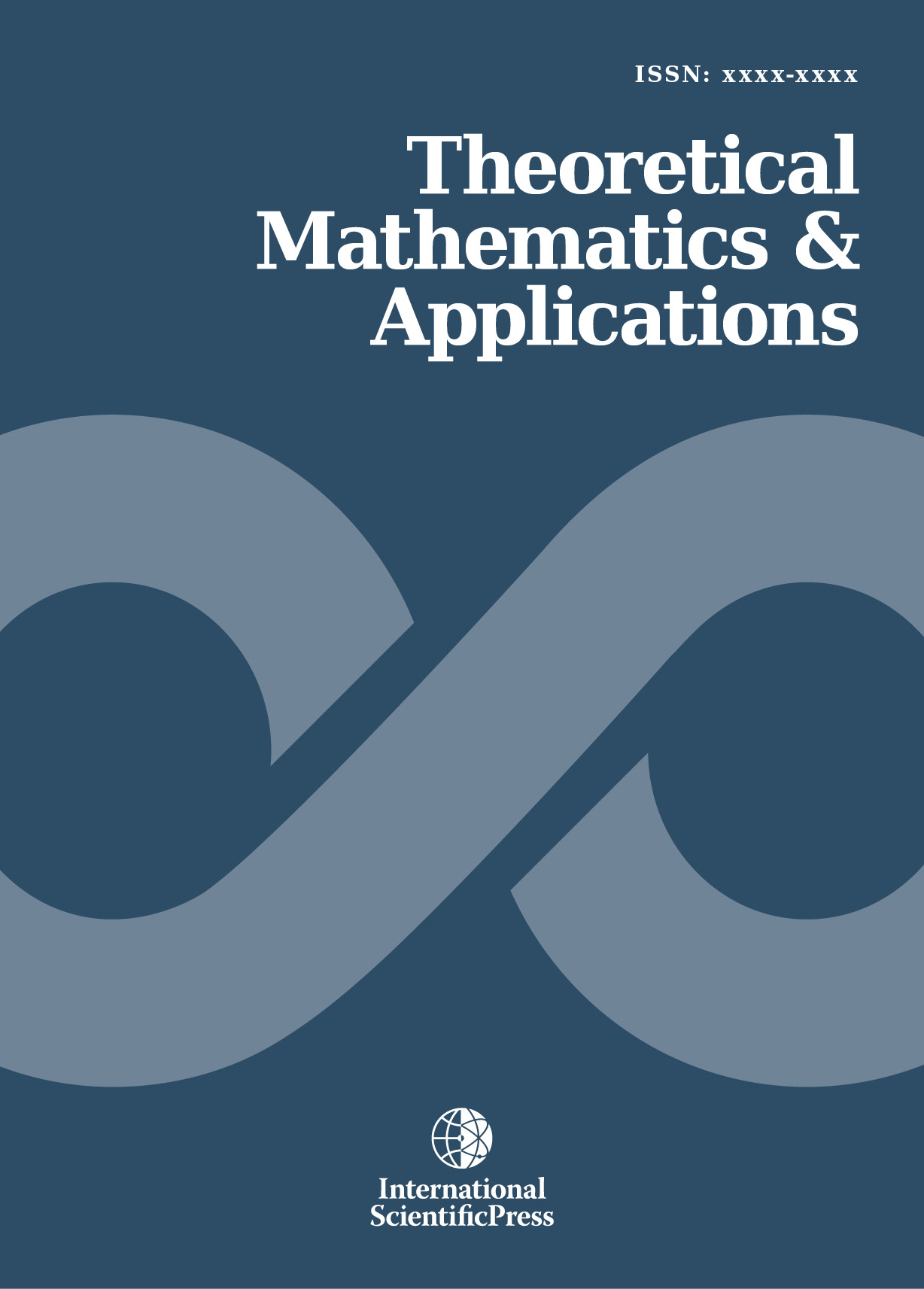# Theoretical Mathematics & Applications

#### Continuity of the quenching time in a nonlinear parabolic equation with a potential

•[ Download ]
• Abstract

In this paper, we consider an initial-boundary value problem. Under some assumptions, we show that the solution of the above problem quenches in a finite time and estimate its quenching time. We also prove the continuity of the quenching time as a function of the initial datum and a potential. Finally, we give some numerical results to illustrate our analysis.

JEL classification numbers: 35B40, 35B50, 35K60, 65M06

Keywords: Quenching, nonlinear parabolic equation, numerical quenching.ISSN: 1792-9687 (Print)
1792-9709 (Online)